| my account | login-logout | resources | classroom help | support | catalog | home | get webcard |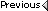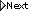» Online Classroom   »   » Public Discussion of Cel Nav   » Navigational Triangle

 Shawn Waldowski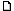posted February 10, 2004 12:14 PM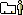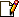I understand one angle is the Assumed Position (AP) and the second angle is the sun or Declination but don't understand why the pole (north or south) is used as the third angle. Your insight is greatly appreciated.Thanks!
 David Burchposted February 12, 2004 12:09 AMThe navigation (spherical) triangle is made up from the great circle arcs on the earth between three points: the assumed position of the observer (AP), the geographical position (GP) of the body being observed, and the elevated pole for the observer... ie north pole for north lat observers, south pole for south lat observers. You could indeed draw and solve the triangle for any observer using any pole, but the above is conventional method and most solutions are based on it. As shown, below, however, you must have some pole in the picture.The intention of the triangle in the first place is to solve for the angular height (Ho) and true bearing (Zn) of a celestial body assuming you know where you are (AP) and you know where the body is (GP).The reason you need one vertex to be a pole is because of the way directions are defined. They are all defined relative to a line from you (AP) to the pole...and ultimately, in the final solution, the reference point is the north pole for both north and south latitudes.If I solve the triganle and get a result, such as Ho = 35° 24.5' and Zn = 223.4° that means that the bearing to the body is 223.4° to the right of the line that joins me (AP) to the North Pole (NP), ie my meridian, which is line [AP to NP].The triangle can be solved with spherical trionometery whenever you know side-angle-side, that is any two sides and the angle between them.Unlike plane trig, where triangles are made of distances on a flat plane and flat angles between them, spherical triangles are made up of all angles. The "sides" are themselves angles, representing an arc on the surface of the earth, and the "angles between the sides" are the angles between these arcs as drawn on the globe.The known quantities of the navigational triangle are side 1 [GP to NP] = 90° - (dec of GP); side 2 [AP to NP] = 90° - (a-lat), and the angle between these two sides is the LHA = (GHA of the GP) - (a-lon), which is the angle between the two sides [NP to AP] and [NP to GP]. We then use this side-angle-side knowledge to solve for z = zenith distance = the arc [AP to GP] and Z = azimuth angle = the angle between the two arcs [AP to NP] and [AP to GP].From z we find Ho = 90° - z and from Z (the bearing of the body relative to the elevated pole, we find Zn, the true azimuth of the body.In other words, the pole is a crucial vertex of the triangle for getting what we want.When i get a chance i will add a picture that will help illustrate these defintions. From: Starpath, Seattle, WA